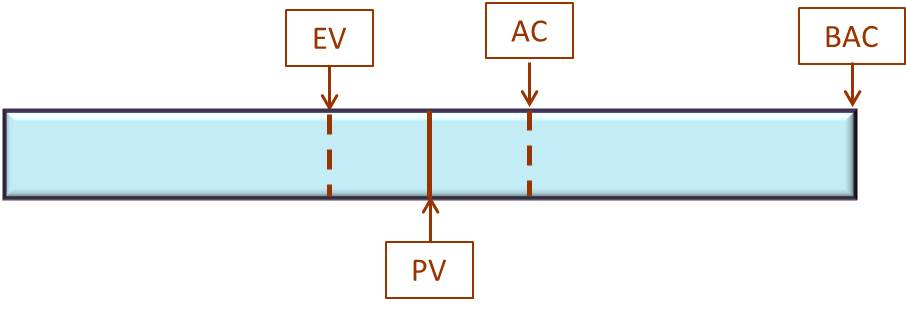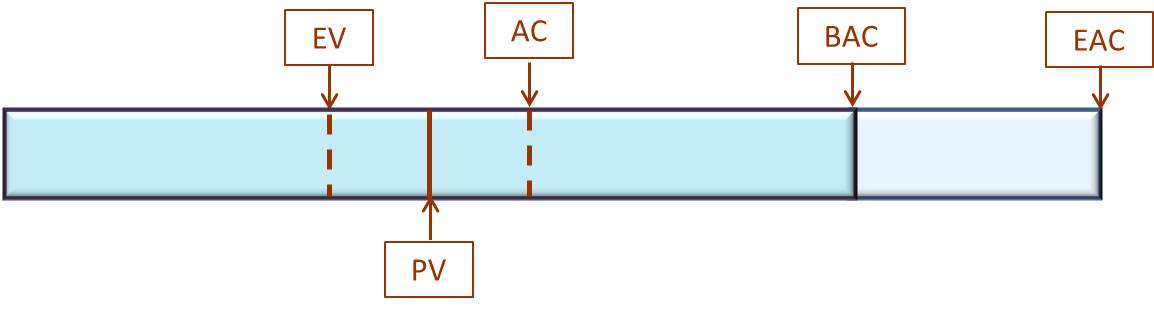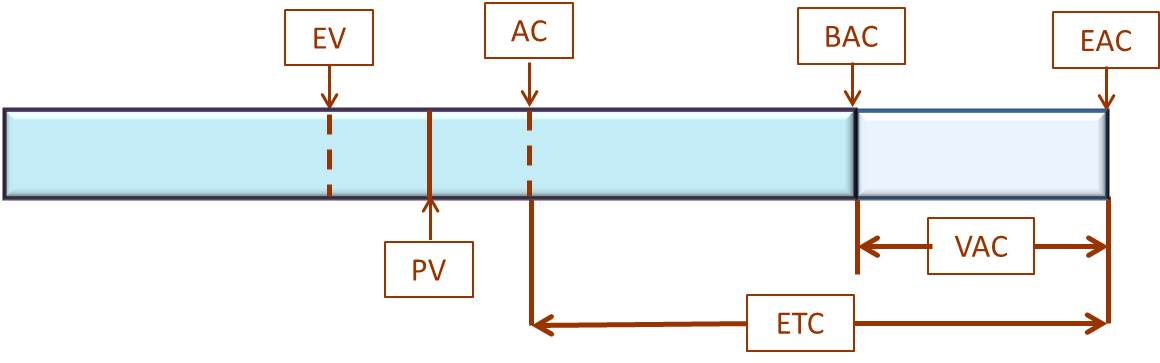# Understanding Earned Value Management: Part 2 – Forecasting a Project

### PMBOK®Guide Definition:

Earned Value Management (EVM): A methodology that combines scope, schedule, and resource measurements to assess project performance and progress.

Actual Cost (AC): The realized cost incurred for the work performed on an activity during a specific time period.

Planned Value (PV): The authorized budget assigned to scheduled work.

Earned Value (EV): The measure of work performed expressed in terms of the budget authorized for that work.

Budget at Completion (BAC): The sum of all budgets established for the work to be performed.

Estimate at Completion (EAC): The expected total cost of completing all work expressed as the sum of the actual cost to date and the estimate to complete.

Estimate to Complete (ETC): The expected cost to finish all the remaining project work.

Variance at Completion (VAC): A projection of the amount of budget deficit or surplus, expressed as the difference between the budget at completion and the estimate at completion.

### Practical Definition:

Earned Value Management (EVM): A set of mathematical formulas for determining current project performance and progress and used to forecast anticipated results; strikes the greatest fear in PMP® aspirants seeking certification.

Actual Cost (AC): The amount of money spent to date on the project.

Planned Value (PV): The planned amount of money to be spent or the amount of progress expected to date on the project.

Earned Value (EV): The value earned or the progress made to date on the project.

Budget at Completion (BAC): The budget value determined after planning the required work for the project.

Estimate at Completion (EAC): The new total budget value calculated during project execution.

Estimate to Complete (ETC): The amount of budget needed from the current point in the project to the new estimated completion.

Variance at Completion (VAC): The difference between the original budget amount and the newly calculated budget needed; the difference between BAC and EAC.

Note: This is the second article in a series describing Earned Value Management (EVM). The first article uses a conceptual model describing the current state of a project. This article uses a conceptual model describing the forecasting of the project. The third article defines the mathematics behind the current project state conceptual model of the first article. The fourth article puts the mathematics behind this article’s conceptual model for forecasting the project. The fifth article provides the bigger picture of Earned Value Management within the context of the overall project.

### Forecasting our Project

Our first article, Part 1 – Understanding Current State of the Project, helped us understand the current state of the project. The next big question to answer is, based on the current project information, how much more money will be needed to complete this project. The example we used, showed we are 50% into the project time frame with the progress around 40% and 60% of the budget spent. The graphic puts the situation into perspective.

Earned Value (EV) defines the progress made on the project (about 40%). Planned Value (PV) shows the time spent on the project (about 50%). Actual Cost (AC) indicates the amount we’ve spent so far (60%) and Budget at Completion (BAC) is the budget for the project – in our case, \$10,000.Earned Value (40%), Planned Value (50%), Actual Cost (60%) and Budget at Completion (\$10,000)

We can see from the graphic, our progress is lagging behind our expected timeline and expenditures. So what should we do? Should we change the scope to stay within the current budget? Do we buy cheaper parts or labor without sacrificing quality? Do we continue beating the team members to increase their productivity and finish on time (the pirate Blackbeard was particularly fond of that method)? Or, should we pull out our forecasting model from the Earned Value Management methodology and re-cast the numbers?

### Earned Value Management Forecasting Concept

We decide it’s best to complete the entire scope of the project using the same parts we originally planned and with the same team members (and we’ve hung up our whips). We must ask for more money and time to accomplish the project. How much more do we need? The Project Sponsor will surely want to know. The picture below shows an additional estimated amount.Depicting Project with progress information, actual costs to date and new estimated total cost

We see the introduction of a new term here – Estimate at Completion (EAC). This is the value determined to be spent in total for the project. In other words, it includes the original budget plus the additional money added to complete the project.

Let’s say the original budget for the home project we are using as our example was \$10,000. Due to some unforeseen circumstances, materials cost more than originally anticipated or simply because we decided to add additional work to the project, we tossed an additional \$5,000 into the money pot. EAC or Estimate at Completion is \$15,000 – the original \$10,000 (BAC) plus the \$5,000. Again, you did this without the benefit of any mathematical formula; you simply estimated the additional amount.

### What’s Estimate to Complete (ETC)

The next question becomes, how much money do we have currently to complete the project? This is known as Estimate to Complete (ETC). It is the remaining money from the original budget plus the amount added. In our example, we have \$4,000 (BAC – AC) from the original estimate plus the \$5,000 added totaling \$9,000. Therefore, we must complete the remaining work on the project using a total of \$9,000. We don’t want to ask for more money. The following picture illustrates the ETC (Estimate to Complete) value.Depicting Project with progress information and listing ETC and VAC

### Variance At Completion

The next variable we calculate is called Variance at Completion (VAC). VAC is simply the difference between our original budget (BAC) and our final budget (or total expenditure – EAC). VAC is expressed in negative terms if EAC is greater than BAC indicating an over-budget condition. If we under spent, VAC is expressed in positive terms.

We forecasted an additional \$5,000 to complete the project. How did we do that? We probably listed additional material we needed plus re-calculated the prices of things purchased which were greater than the original estimate. If you are like us, when it comes to home projects, we “eyeballed” it and came up with a number (“back of the envelope” calculation method).  Nothing scientific, but good enough. Of course, that would never work for our  project sponsor, especially since the VAC will probably be more than \$5,000.  We will have to revert to using the Earned Value Management formulas, which we’ll cover in the next article.

### To Complete Performance Index

A final calculation we can use is “To Complete Performance Index.” Let’s say, when we decided to add a measely \$5,000 to our original budget to complete the ultimate man-cave, the spouse simply said, “NO!” We decided to heed the advice and not put in the extra galley sink, baby refrig or bigger screen TV and just go with the original plan. In fact, the “NO!” meant we had to stay within our original budget. Based on that feedback, we need to calculate a new spend rate or cost performance to complete the scope within the remaining budget. In other words, what did we need to improve our current Cost Performance Index (CPI) to not require more money. That calculated value is To Complete Performance Index (TCPI).

### Conclusion

In this article, we developed the concept of predicting future needs for our project. We used a conceptual model to illustrate the basis for the equations to be described in the next article where we’ll explore the mathematics of EVM. Having this basis of understanding puts a context around the functioning of the formulas.

In the next article, we will list each of the formulas necessary to know for the Project Management Professional (PMP®) certification exam. We continue to use our illustrations tying the mathematics back to the concept. We have learned from teaching thousands of students a picture is worth a thousand iterations of memorizing abstract formulas. Seeing how they are derived helps recalling the formula while taking the exam.